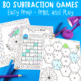# Subtraction Strategies for Number Fact Fluency | Distance LearningSubject
Resource Type
File Type
Zip (97 MB|114 pages)
\$7.50
Also included in:
1. Knowing and applying addition strategies and subtraction strategies help improve fact fluency. We all want our young learners to confidently answer their number facts with no hesitating or counting on fingers. To be able to fluently add or subtract within twenty using mental math strategies and know
\$15.00
\$12.50
Save \$2.50
2. Knowing and applying addition strategies and subtraction strategies help improve fact fluency. We all want our young learners to confidently answer their number facts with no hesitating or counting on fingers. To be able to fluently add or subtract within twenty using mental math strategies and know
\$31.00
\$22.00
Save \$9.00
Product Description

Subtraction fact fluency – we all want our young learners to confidently answer their subtraction facts with no hesitating or counting on fingers. To be able to fluently subtract within twenty using mental strategies is math standard for all second graders.

You can help them become fluent and increase their confidence in math by teaching them the subtraction strategies that go beyond just relating subtraction to addition facts.

Distance Learning

This pack of 55 single player or partner games and activities is perfect for teachers organizing distance learning or parents looking for at home learning activities.

(⭐ Looking for addition strategy games specifically for Google Classroom? Try Subtraction Strategies Distance Learning pack 1 or Subtraction Strategies Distance Learning pack 2)

This math unit contains:

⇒ Teacher explanation for each strategy.

⇒ A Flip book - use a reference tool for when the class is learning each subtraction strategy.

⇒ 5 activities (a total of 55 activities) which can be used independently or in pairs for consolidating each of the following strategies:

- Subtract from 10

- Counting back 1

- Counting back 2

- Counting back 3

- Counting back 1, 2 and 3

- Count Up

- Subtracting 0

- Doubles Subtraction

- Near Doubles Subtraction

- Subtracting 9

- Subtracting 10

⇒ 5 activities (a total of 25 activities) which can be used independently or in pairs to consolidate the strategies when ...

- Subtracting 4

- Subtracting 5

- Subtracting 6

- Subtracting 7

- Subtracting 8

⇒ Interactive workbook pockets for using the above strategies. Students answer the number facts and sort them into the strategy pockets.

(British English is included.)

It's the perfect companion to Addition Strategies for Number Fact Fluency

You might be interested in more games and activities using subtraction strategies:

Subtraction Strategy Games

Subtraction Strategies - Homework Games

Fact Families Card Sort

Become a follower and take advantage of my Early Bird Specials...all new products, excluding bundles, will be 50% off for the first 24 hours after being listed.

Total Pages
114 pages